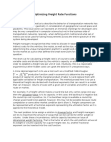# Optimizing Freight Rate Functions

By Alfonso LlanesUsing discrete mathematics to describe the behavior of transportation networks has to include Riemannian geometry in consideration of geographical curved space and gradients. This issue alone can represent an immense challenge for developers who may be very competitive in computer science but not in the business side of transportation networks. Specially, when defining which method and what set of metrics are to be used for taking measurements across the entire spectrum of the system being developed. Freight managers recognize that they need to allocate in separate sets direct and indirect costs for the vehicles, the routes, as well as the size, and capacity, for determining the unique transportation platform's weight and cubic space available for the market at a price that defines the break-even point for a specific case in question. The short cut for calculating a freight rate is of course to add all the fixed and variable costs and divide them by the vehicle's capacity on either weight or space in order to establish a freight rate per unit of cost. Intuitively, this is a reasonable argument but other hidden costs can upset the balance in unexpected ways. A more labored approach is to use a tool such as a modified Cobb-Douglas production function used in economics to determine the marginal product of capital (K) and the marginal product of labor (L) and replace them with applicable variables for freight transportation. The method prosed here is to use a theoretical freight rate divided with fractional exponents that can result in a model that can measure returns of scale with a given set of variables and within a specific route or market. For example, if a freight vehicle makes a round trip but only carries cargo one way then the utilization factor is ½ its capacity. In this case, the backhaul is negative but the round trip cost is positive. So, if no backhaul cargo is available a round trip charge would need to be applied, however, this may not be possible because route competition or some other market condition don't allow it. Freight component can be represented with a fractional exponent representing the utilization factor as it is applied to the theoretical model. The next problem to be assign a solution is stowage factor and freight managers will try to maximize the amount of cargo that can be carried for either weight or volume. Under these circumstances, vehicle capacity becomes an issue of dimensional weight factoring. That is the factor at which the unit platform arrives at its weight or volume capacity for a given freight rate. So, another fractional exponent can be inserted into the cost function. Determining maximum carrying value on a limited transportation platform by either weight or volume is an issue that can, in many cases, determine success or failure of a transportation company. The theory behind the problem has been endlessly studied with " the knapsack problem " which, relates to combinatorial optimization between weight, space and value. Given a set of cargoes, each with a weight, a volume and value, freight managers must determine the number of each unit that can be included in a collection of items equal to a given limit where the total commodity-freight-value is as large as possible. Thus, the problem becomes an issue of optimizing a vehicle utilization factor for any given trip. As a result, another fractional exponent representing commodity value can be added to the function.